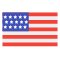enStyles
Categories
Trends
Old Interface
CollectionsView All
Drag icons
here

# Not equal Icons

Unequal
Collection
Unequal
Collection
Unequal
Collection
Unequal
Collection
Unequal
Collection
Unequal
Collection
Unequal
Collection
Unequal
Collection
Unequal
Collection
Approximately Not Equal
Collection
Approximately Not Equal
Collection
Approximately Not Equal
Collection
Approximately Not Equal
Collection
Approximately Not Equal
Collection
Approximately Not Equal
Collection
Approximately Not Equal
Collection
Approximately Not Equal
Collection
Approximately Not Equal
Collection
Approximately Not Equal
Collection
Approximately Not Equal
Collection
Approximately Not Equal
Collection
Approximately Not Equal
Collection
Approximately Not Equal
Collection
Approximately Not Equal
Collection
Approximately Not Equal
Collection
Approximately Not Equal
Collection
Approximately Not Equal
Collection
Approximately Not Equal
Collection
Approximately Not Equal
Collection
Approximately Not Equal
Collection
Approximately Not Equal
Collection
Approximately Not Equal
Collection
Approximately Not Equal
Collection
Approximately Not Equal
Collection
Approximately Not Equal
Collection
Approximately Not Equal
Collection
Approximately Not Equal
Collection
Approximately Not Equal
Collection
Approximately Not Equal
Collection
Approximately Not Equal
Collection
Approximately Not Equal
Collection
Approximately Not Equal
Collection
More or Equal
Collection
More or Equal
Collection
More or Equal
Collection
More or Equal
Collection
More or Equal
Collection
More or Equal
Collection
More or Equal
Collection
More or Equal
Collection
More or Equal
Collection
More or Equal
Collection
More or Equal
Collection
More or Equal
Collection
More or Equal
Collection
More or Equal
Collection
More or Equal
Collection
More or Equal
Collection
More or Equal
Collection
More or Equal
Collection
More or Equal
Collection
Less or Equal
Collection
Less or Equal
Collection
Less or Equal
Collection
Less or Equal
Collection
Less or Equal
Collection
Less or Equal
Collection
Less or Equal
Collection
Less or Equal
Collection
Less or Equal
Collection
Less or Equal
Collection
Less or Equal
Collection
Less or Equal
Collection
Less or Equal
Collection
Less or Equal
Collection
Less or Equal
Collection
Less or Equal
Collection
Less or Equal
Collection
Less or Equal
Collection
Less or Equal
Collection
Equal Sign
Collection
Equal Sign
Collection
Equal Sign
Collection
Equal Sign
Collection
Equal Sign
Collection
Equal Sign
Collection
Equal Sign
Collection
Equal Sign
Collection
Equal Sign
Collection
Equal Sign
Collection
Equal Sign
Collection
Equal Sign
Collection
Equal Sign
Collection
Equal Sign
Collection
Equal Sign
Collection
Equal Sign
Collection
Equal Sign
Collection
Equal Sign
Collection
Equal Sign
Collection
Equal Sign
Collection
Equal Sign
Collection
Less or Equal
Collection
Less or Equal
Collection
Less or Equal
Collection
Less or Equal
Collection
Less or Equal
Collection
Less or Equal
Collection
Less or Equal
Collection
Less or Equal
Collection
Less or Equal
Collection
Less or Equal
Collection
Less or Equal
Collection
Less or Equal
Collection
Less or Equal
Collection
Less or Equal
Collection
Less or Equal
Collection
Less or Equal
Collection
Less or Equal
Collection
Less or Equal
Collection
ID not Verified
Collection
Collections
0 set
Create new
Drag icons here